Financial Accounting Lecture 17 Fixed Assets Fixed assets

• Slides: 21Financial Accounting Lecture – 17 Fixed Assets • Fixed assets are those assets: n n n that have a long life, are used in the business for future generation of income, are not bought with the main purpose of resale. 1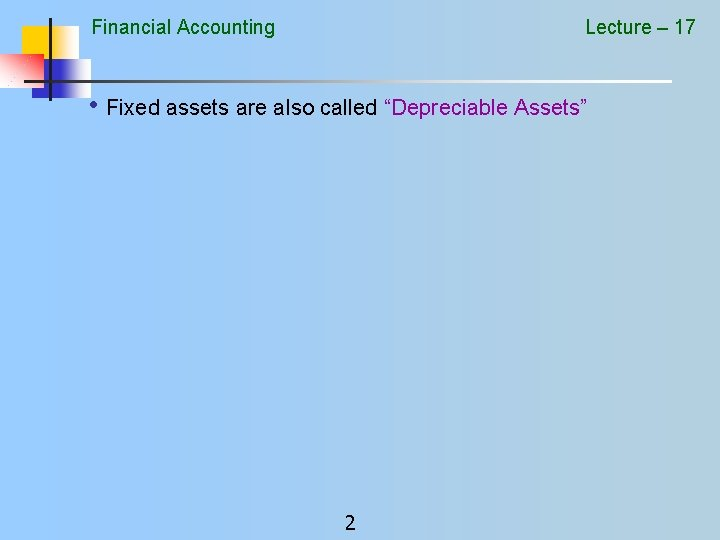Financial Accounting Lecture – 17 • Fixed assets are also called “Depreciable Assets” 2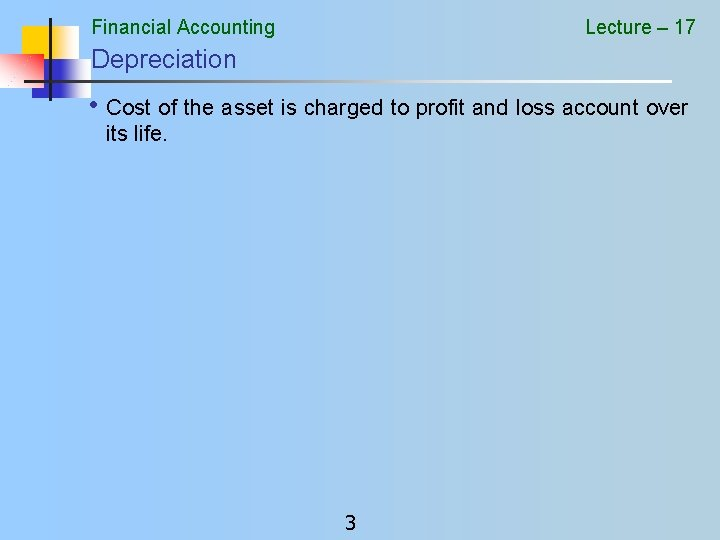Financial Accounting Lecture – 17 Depreciation • Cost of the asset is charged to profit and loss account over its life. 3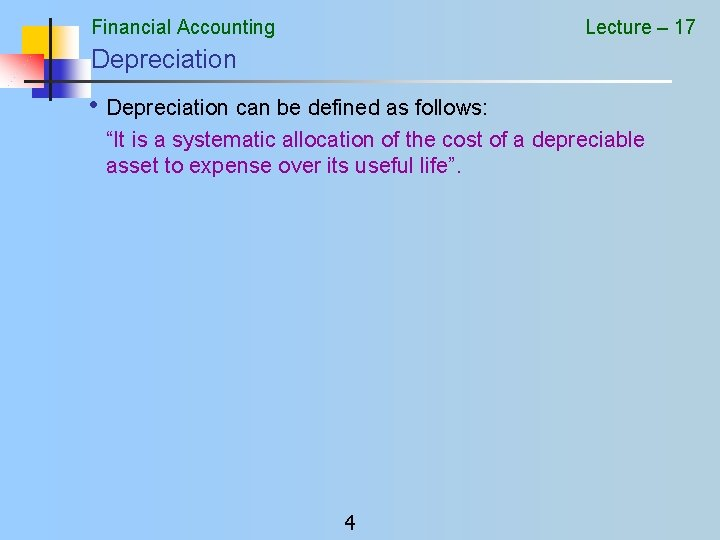Financial Accounting Lecture – 17 Depreciation • Depreciation can be defined as follows: “It is a systematic allocation of the cost of a depreciable asset to expense over its useful life”. 4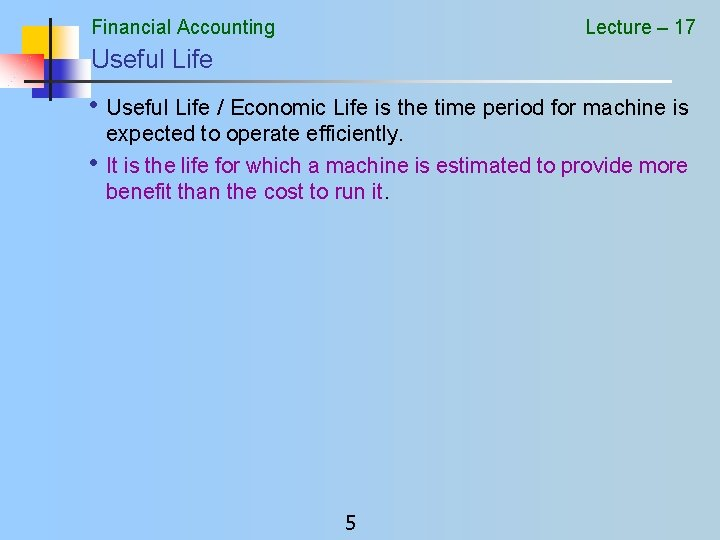Financial Accounting Lecture – 17 Useful Life • Useful Life / Economic Life is the time period for machine is • expected to operate efficiently. It is the life for which a machine is estimated to provide more benefit than the cost to run it. 5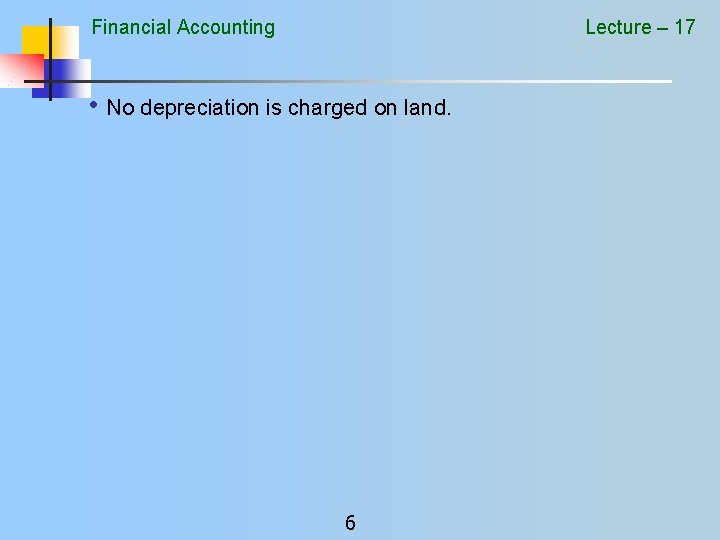Financial Accounting Lecture – 17 • No depreciation is charged on land. 6Financial Accounting Lecture – 17 • In case of Leased / Lease Hold Land the amount paid for it is charged over the Life of Lease. • It is called Amortization. 7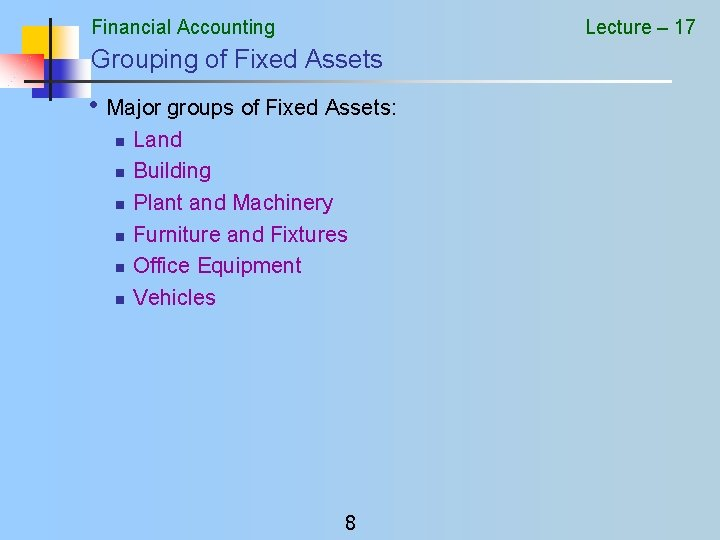Financial Accounting Lecture – 17 Grouping of Fixed Assets • Major groups of Fixed Assets: n n n Land Building Plant and Machinery Furniture and Fixtures Office Equipment Vehicles 8Financial Accounting Lecture – 17 Recording • Purchase of a Fixed Asset Debit Credit Account Asset Account (relevant classification) Cash / Bank or Payable 9Financial Accounting Lecture – 17 Recording Depreciation • Two different accounts are used n Depreciation Expense Account n Accumulated Depreciation Account 10Financial Accounting Lecture – 17 Accumulated Depreciation Account – over the years the periodic depreciation is accumulated in this account. 11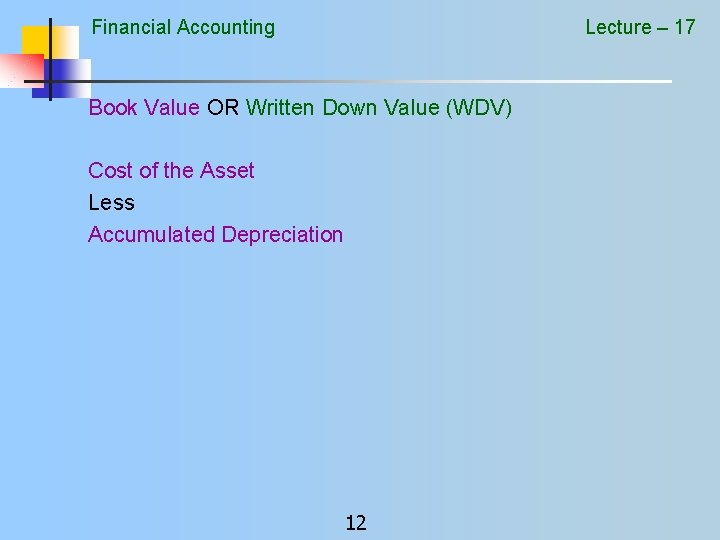Financial Accounting Lecture – 17 Book Value OR Written Down Value (WDV) Cost of the Asset Less Accumulated Depreciation 12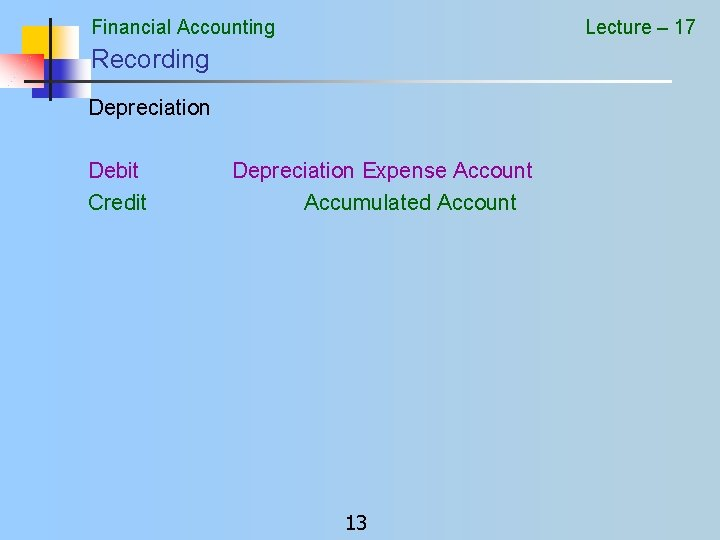Financial Accounting Lecture – 17 Recording Depreciation Debit Credit Depreciation Expense Account Accumulated Account 13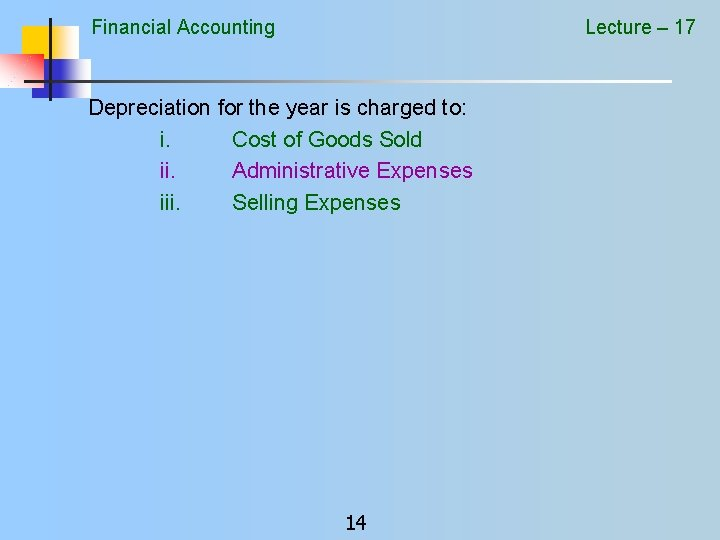Financial Accounting Lecture – 17 Depreciation for the year is charged to: i. Cost of Goods Sold ii. Administrative Expenses iii. Selling Expenses 14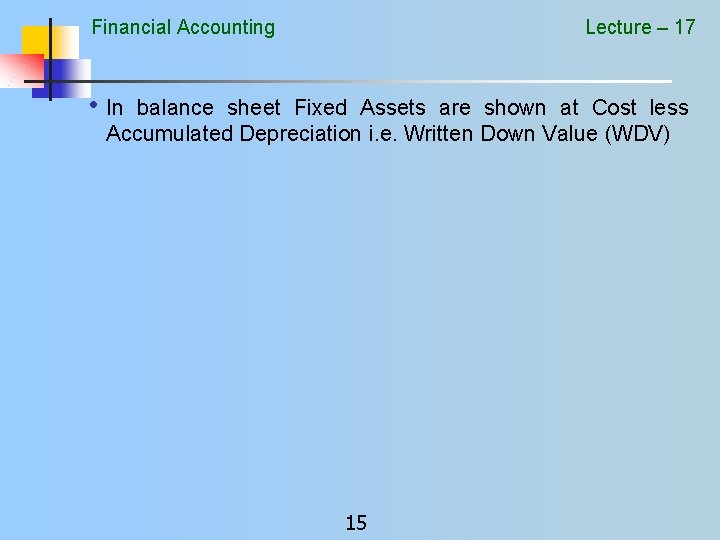Financial Accounting Lecture – 17 • In balance sheet Fixed Assets are shown at Cost less Accumulated Depreciation i. e. Written Down Value (WDV) 15Financial Accounting Lecture – 17 Recording the Depreciation • Journal Entry • • • Debit Depreciation Expense Account Credit Accumulated Depreciation Account Presentation Profit and Loss Account Revenue - Cost of Sales - Admin, Selling and Financial Expenses Balance Sheet Fixed Assets - Accumulated Depreciation 16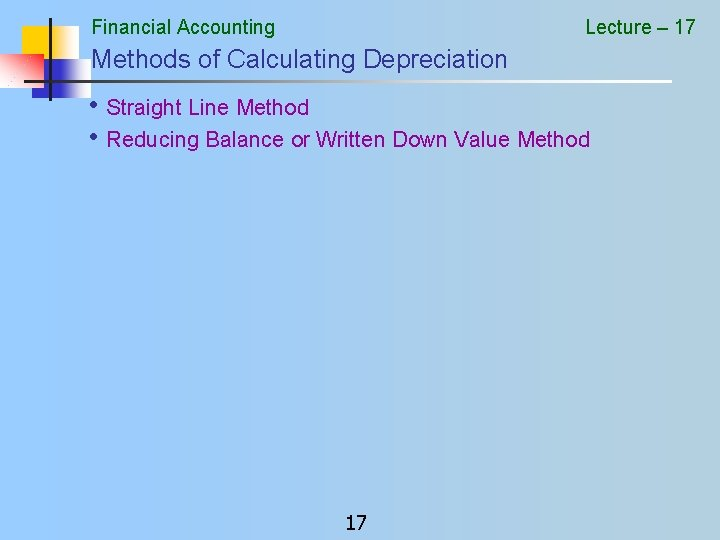Financial Accounting Lecture – 17 Methods of Calculating Depreciation • Straight Line Method • Reducing Balance or Written Down Value Method 17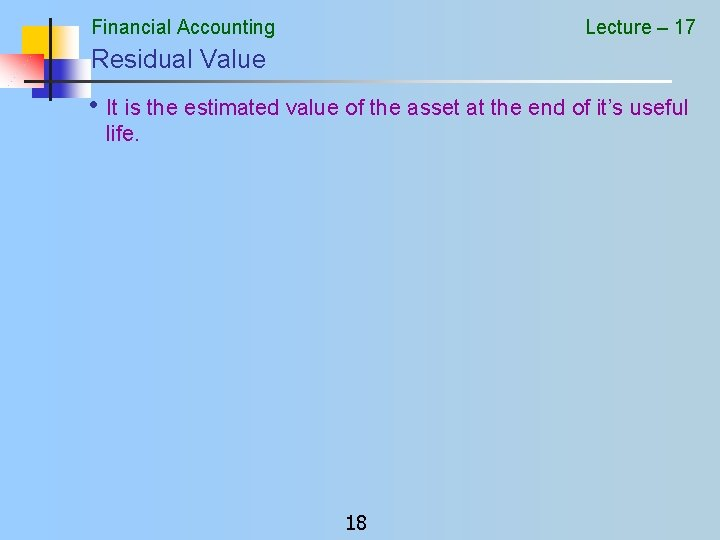Financial Accounting Lecture – 17 Residual Value • It is the estimated value of the asset at the end of it’s useful life. 18Financial Accounting Lecture – 17 Straight Line Method of Calculating Depreciation = (Cost – Residual Value) / Life of The Asset 19Financial Accounting Lecture – 17 Example Straight Line Method • Cost of the Asset • Life of the Asset • Annual Depreciation = Rs. 100, 000 = 5 years = 20 % of cost or Rs. 20, 000 20Financial Accounting Lecture – 17 Written Down Value Method • Cost of the Asset • Annual Depreciation n n = Rs. 100, 000 = 20% = 20 % of 100, 000 = 20, 000 = 100, 000 – 20, 000 = Year 1 Depreciation Year 1 WDV 80, 000 Year 2 Depreciation 16, 000 Year 2 WDV 64, 000 = 20 % of 80, 000 = 80, 000 – 16, 000 21 = =